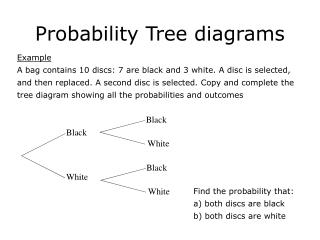DownloadDownload PresentationProbability Tree diagrams

# Probability Tree diagrams

Download Presentation## Probability Tree diagrams

- - - - - - - - - - - - - - - - - - - - - - - - - - - E N D - - - - - - - - - - - - - - - - - - - - - - - - - - -
##### Presentation Transcript

1. Black Black White Black White White Probability Tree diagrams Example A bag contains 10 discs: 7 are black and 3 white. A disc is selected, and then replaced. A second disc is selected. Copy and complete the tree diagram showing all the probabilities and outcomes Find the probability that: a) both discs are black b) both discs are white

2. three three Not a 3 three Not a 3 Not a 3 Example A six sided dice is thrown twice. Complete the tree diagram showing all the probabilities. What is the probability of throwing a total of (i) two threes? (ii) No threes? (iii) At least one three?

3. Example A bag contains 5 red balls and 3 green balls. A ball is drawn at random and then replaced. Another ball is drawn. What is the probability that both balls are green?

4. Vowel Vowel No Vowel Vowel No Vowel No Vowel Example The letters of the word ‘INDEPENDENT’ are written on individual cards and the cards are put into a box. A card is selected and then a second card is selected. Copy and complete the tree diagram below Find the probability of obtaining: a) One vowel b) two vowels

5. Example • A bag contains 4 red balls and 2 green balls. A ball is drawn and not replaced. A second ball is then selected. • Draw tree diagram to show all possible outcomes. • Find the probability of selecting one red and one green ball in • any order.

6. Example A card is selected from a pack of 52 playing cards. The card is then replaced and a second card is selected. What is the probability of selecting: (i) a heart for the first card? (ii) Two hearts?

7.  Example A weather forecast says The probability that it will rain on Monday is The probability that it will rain on Tuesday is  (a) Complete the tree diagram showing the possible outcomes (b) Calculate the probability that it rains on just one of the two days

8. Example The diagram shows a spinner. When the arrow is spun the probability of scoring 2 is The arrow is spun twice and the scores are added Complete the tree diagram and work out the probability of scoring a 4. First Spin Second spin 2 2 3 2 3 3

9. Example • At the end of a training programme students have to pass an exam to gain a certificate.The probability of passing the exam at the first attempt is 0.75Those who fail are allowed to re-sit.The probability of passing the re-sit is 0.6 • No further attempts are allowed. • Complete the tree diagram below showing all the possible outcomes

10. What is the probability that a student fails to gain a certificate? • Three students take the exam.What is the probability that all of them gain a certificate?

11. Example • Greg has a fair spinner with 5 equal sections.Three sections are red and two are blue.He spins the spinner twice. • draw the probability tree diagram to show the possible outcomes. • Calculate the probability that the spinner lands on red both times.

12. Example • Sally and Susannah take turns to throw a dart at a given target.The probability that Sally hits the target is 0.4The probability that Susannah hits the target is 0.2 • draw the tree diagram • What is the probability that Sally and Susannah both hit the target ?